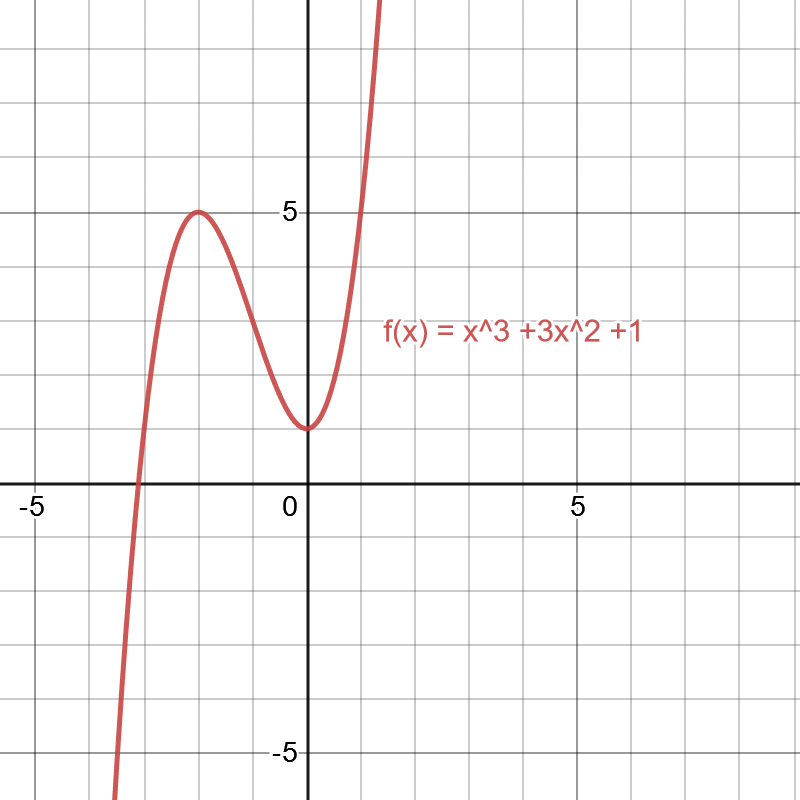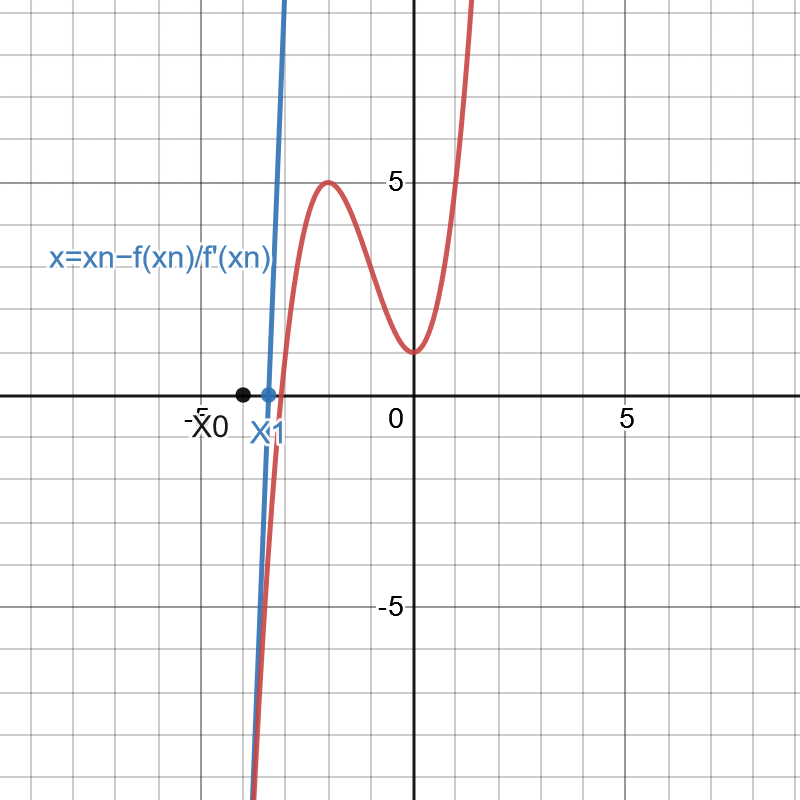As systems of nonlinear equations becomes more complex, it will be harder to obtain solutions by substitutions or graphing. Newton’s method allows us to quickly solve for the system. It is also the method that we will implement in solving using NMdev.

What is Newton’s Method?

The newton’s method, also known as the Newton–Raphson method, is a root-finding algorithm which produces successively closer approximations to the actual roots of a real-valued function. The basic concept is to start with an initial guess which is close to the true root,$x_{0}$, then to approximate the function by its tangents, and then compute the x-intercept of this tangent line. Suppose we need to find the roots this function$f$, where the equation of the graph is$f(x)=x^3 +3x^2+1$From the graph, a good close initial guess would be -4. With that, we can draw the tangent line and find the x-intercept of the tangent. This x-intercept will typically be a better approximation to the original function’s root than the first guess. By Taylor series expansion,$f(x)=f(a)+f^{\prime}(a)(x-a)+\frac{f^{\prime\prime}(a)(x-a)^{2}}{2}+$ . . .

We can substitute in$x$ as the solution and$a$ as the guess,$x_{0}$.$f(x)=f(x_{0})+f^{\prime}(x_{0})(x-x_{0})+\frac{f^{\prime\prime}(x_{0})(x-x_{0})^2}{2}+$ . . .

If$x_{0}$ is sufficiently close to$x$,$(x_{0}-x)$ is small, and higher powers of$(x_{0}-x)$ can be ignored. We can then rewrite the equation, substituting x  as x1 which is the value of x which is closer to the solution, and let f(x)=0 since we are finding the roots where y=0.$0=f(x_{0})+f'(x_{0})(x_{1}-x_{0})$

Solving for x1 gives$f'(x_{0})(x_{1})=f'(x_{0})(x_{0})-f(x_{0})$$x_{1}=x_{0}-\frac{f(x_{0})}{f'(x_{0})}$

The equation can be rewritten in a general form as a iterative equation,$x_{n+1}=x_{n}-\frac{f(x_{n})}{f'(x_{n})}$

where xn+1 is a value that is more accurate than xn, provided this initial guess is close enough to the unknown zero, and that f ′(x0) ≠ 0.Using this equation of the graph, we are now able to deduce the value of x1$x_{1}=-4-f(-4)/(f'(-4))$$f(-4)=(-4)^3+3(-4)^2+1=-15$$f'(-4)=3(-4)^2+6(-4)+1=25$$x_{1}=-4+15/25=-3.4$

The idea behind Newton’s system is that each x-intercept that we find with our tangent will be closer to the root than our initial guess. Graphically, when we plot the values and the tangent of x0 on the graph, we will find that the new x-intercept, x1, of this tangent is closer to the root than the initial value of x that we guessed.We can then continue to repeat this process iteratively until we arrive at a value that is precise enough. Continuing with the process using x1, we can obtain the value x2.$x_{1}=-3.4-f(-3.4)/(f'(-3.4))$$f(-3.4)=(-3.4)^3+3(-3.4)^2+1=-3.624$$f'(-3.4)=3(-3.4)^2+6(-3.4)+1=15.28$$x_{1}=-3.4+3.624/15.28=-3.1628$

Graphically, we can show that x2 is closer to x1 by plotting the tangent at x=x1.We will keep iterating Newton’s method until the precision threshold is satisfied or the maximum number of iterations is reached. The difference of x to the actual root by the time the precision threshold is satisfied should be negligible, and hence with Newton’s root method, we are able to find the roots of a nonlinear equation. In the next chapter, we will look at how to apply Newton’s method to a system of non-linear equations, as well as how use the NewtonSystemRoot class in NMdev to implement a solver for systems of non-linera equations.